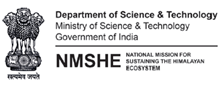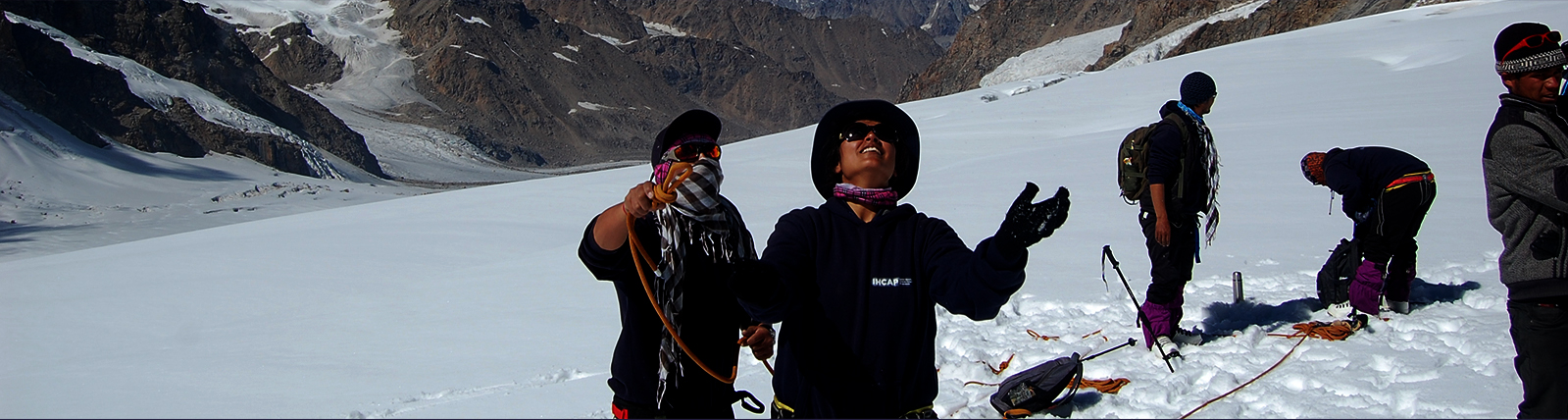## Glacier mass balance approaches and methods

The course provides a basic understanding of glacier mass budget, glacier mass balance gradient and glacier mass balance profile. The course describes in detail the different glacier mass balance methods including: Direct/Glaciological method, Geodetic, Hydrological and AAR based method, limitations and strengths. The course also gives an overview of glacier mass balance modelling approaches: Temperature Index modelling, energy balance modelling and linear mass balance modelling.

• Summary
• Presentation
• Literature
• ExerciseSummary glacier methodsSummary mb modeling approachesintro mb models handoutsintro mb models slidesmb Findelen handoutsmb Findelen slidesmelt modelling handoutsmelt modelling slides